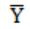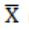## Recursive-Enumerable-Languages

 Question 1

Let X be a recursive language and Y be a recursively enumerable but not recursive language. Let W and Z be two languages such thatreduces to W, and Z reduces to(reduction means the standard many-one reduction). Which one of the following statements is TRUE?

 A W can be recursively enumerable and Z is recursive. B W can be recursive and Z is recursively enumerable. C W is not recursively enumerable and Z is recursive. D W is not recursively enumerable and Z is not recursive.
Theory-of-Computation       Recursive-Enumerable-Languages       GATE 2016 [Set-1]       Video-Explanation
Question 1 Explanation:
The rules are:
If A ≤ p B
Rule 1: If B is recursive then A is recursive
Rule 2: If B is recursively enumerable then A is recursively enumerable
Rule 3: If A is not recursively enumerable then B is not recursively enumerable
Since X is recursive and recursive language is closed under compliment, so is also recursive.
: By rule 3, W is not recursively enumerable.
: By rule 1, Z is recursive.
Hence, W is not recursively enumerable and Z is recursive.
There is 1 question to complete.

Register Now